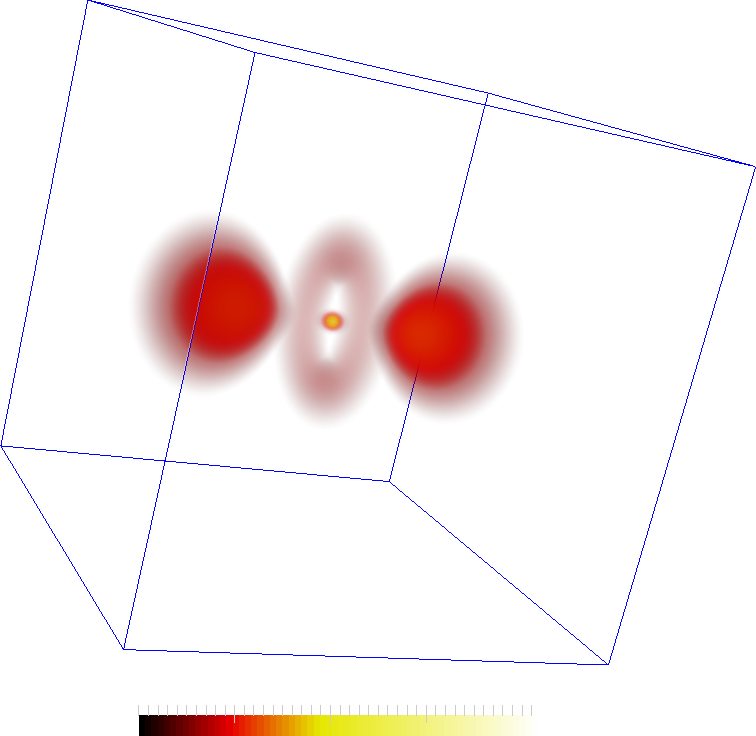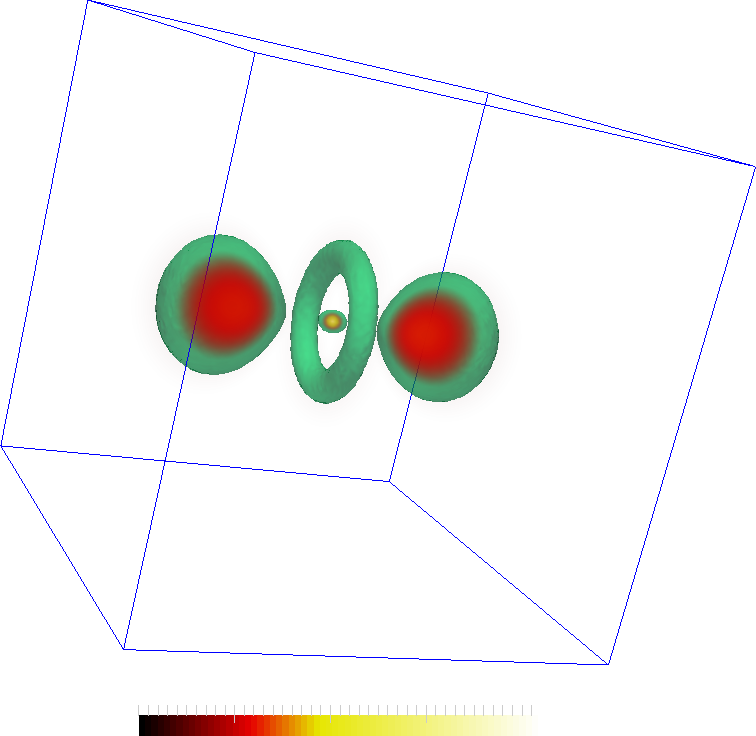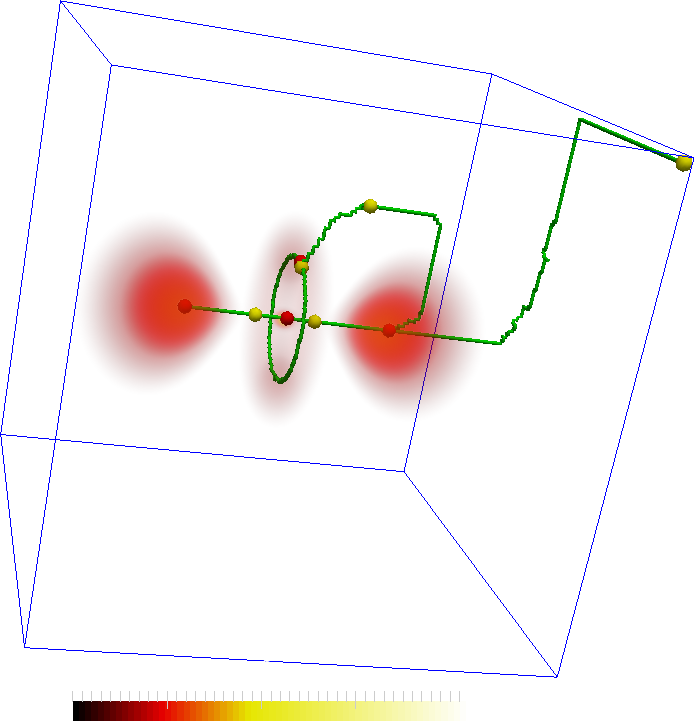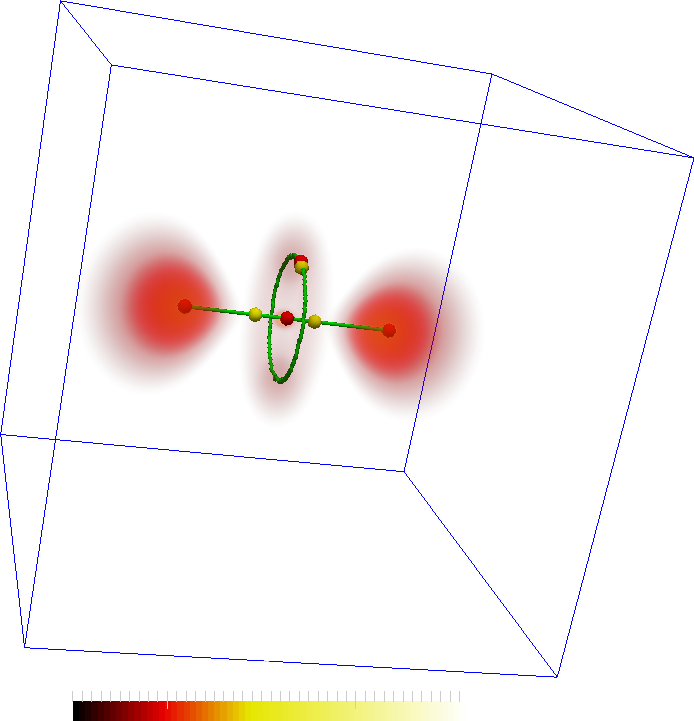## Examples using pyms3d

Here, two case studies presenting how the pyms3d may be plugged into paraview are discussed. The first involves the Hydrogen dataset shown here . The second is the isabel case study and is shown using a video here .

## Hydrogen

The hydrogen dataset is an EM scan of the hydrogen atom available from here . These images shows a simple voume rendering of the scalar values and features extracted from them. The second image also shows an iso-surface at 30. The features are the two lobes, central torus and a maximum in the center of the torus.The features are represented by the maxima (red spheres) the following two images. The connectivity in the Morse-Smale complex captures the torus as well as the connectivity between the central lobes and the central maxima. However, not all connections may be interesting. In the second figure, two saddles are removed so that the remaining correspond more closely to the features in the dataset.An important aspect is the programmable and interactive aspect of the design. The programmable aspect comes in handy when some filtering, such as those for the images above, is required.

The following are a pair of scripts that generate the geometry for the above visualizations. They are to be plugged into the paraview programmable source, and programmable filter respectively. The first script computes the Morse-Smale complex, simplifies it and then generates the geometry of the ascending manifolds of 2-saddles. The second generates the set of critical points that represent this geometry, namely the 2-saddles and the maxima they connect. In the first script, the set of saddles may be trimmed as desired. The line to do this is commented in the first script. It is uncommented to produce the fourth image above. As can be seen in both scripts, the code attempts to load data from a cache and only recomputes if it is unavailable.

The full paraview state file containing the pipeline necessary to generate the above images is available here . The raw float data (8Mb) is available here . Note: the scripts and the paraview state file assumes that the data is available in a folder named data. Edit that appropriately. Also, this was known to work with Paraview 4.3. In general, the Paraview Python API evolves quickly and the code may not work properly on earlier versions.

• ### Programmable Source to generate the 2-saddle geometry

```import pyms3d
from paraview.vtk.numpy_interface import dataset_adapter as DA
import numpy as np

#data info
DataFile = "data/Hydrogen_128x128x128.raw"
Dim      = (128,128,128)

# try to load from cache
if hasattr(pyms3d,"msc") and hasattr(pyms3d,"pa"):
msc  = pyms3d.msc
pa   = pyms3d.pa
else:
# compute the mscomplex simplify and collect the
# ascending geometry of 2 saddles
print pyms3d.get_hw_info()
msc = pyms3d.mscomplex()
msc.compute_bin(DataFile,Dim)
msc.simplify_pers(thresh=0.05)
msc.collect_geom(dim=2,dir=1)

# the ascending geometry of 2-saddles is defined
# on the dual grid. Get coorinates of dual points.
# i.e. The centroids of cubes
dp = msc.dual_points()
pa = vtk.vtkPoints()
pa.SetData(DA.numpyTovtkDataArray(dp,"Pts"))

# Cache objects
setattr(pyms3d,"msc",msc)
setattr(pyms3d,"pa",pa)

#put the list in cache

# create a vtk CellArray for the line segments
ca = vtk.vtkCellArray()
gm = msc.asc_geom(s)
for a,b in gm:
ca.InsertNextCell(2)
ca.InsertCellPoint(a)
ca.InsertCellPoint(b)

#Set the outputs
pd = self.GetOutput()
pd.SetPoints(pa)
pd.SetLines(ca)
```
• ### Programmable Filter to extract the location of 2-saddles and maxima

```import pyms3d
import numpy as np

msc      = pyms3d.msc

# create the list of 2-saddles and maxima locations
cpList = []
cpList.append((msc.cp_cellid(s),2))
for m,k in msc.asc(s):
cpList.append((msc.cp_cellid(m),3))
cpList = list(set(cpList))

# create the vtk objects
pa = vtk.vtkPoints()
ia = vtk.vtkIntArray()
ca = vtk.vtkCellArray()
ia.SetName("Index")

for i,(p,idx) in enumerate(cpList):
pa.InsertNextPoint(np.array(p,np.float32)/2)
ia.InsertNextValue(idx)
ca.InsertNextCell(1)
ca.InsertCellPoint(i)

#Set the outputs
pd = self.GetOutput()
pd.SetPoints(pa)
pd.SetCells(vtk.VTK_VERTEX,ca)